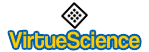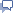Sponsored Question: Daily Deals on eBay or Daily Deals on Amazon??# Properties of Number

Click on number to see Concepts connected with it.
 0 Can be partitioned 1 way. n/a

 1 Can be partitioned 1 way. n/a

 2 Can be partitioned 2 ways. Prime The 3th Fibonacci Number. The 1st Mersenne prime number.

 3 Can be partitioned 3 ways. Prime The 2nd mersenne number. triangular number The 4th Fibonacci Number. The 1st Delannoy Number.

 4 Can be partitioned 5 ways. 2x2 Square Number

 5 Can be partitioned 7 ways. Prime The 8th Fibonacci Number. The 3rd catalan number.

 6 Can be partitioned 11 ways. 2x3 The 3rd triangular number. The 2nd hexagonal number. The 2nd Schr�der number.

 7 Can be partitioned 15 ways. The 4th prime number. The 3rd Mersenne prime number. The 4th mersenne number. The 4th odd number. The 4th Lucas number. The 3rd lucky number. Sum of the first 4 Fibonacci numbers= 1 + 1 + 2 + 3 = 7 Difference of the first two cube numbers = 23 - 13 = 8 - 1 = 7 Sum of the 2nd odd & 2nd even numbers = 3 + 4 = 7 Sum of the 1st & 3rd prime numbers= 2 + 5 = 7 Sum of the 1st & 3rd factorials= 1 + 6 = 7 Sum of the 1st & 3rd triangular numbers= 1 + 6 = 7

 8 Can be partitioned 22 ways. 2x2x2 The 5th Fibonacci Number.

 9 Can be partitioned 30 ways. 3x3 The 5th odd number. The 4th composite number. The 2nd octagonal pyramidal number. (sum of 1st two octagonal #s = 1+ 8 = 9) The 4th lucky number. Sum of the first two cube numbers= 1 + 8 = 9 Sum of the first three odd numbers. Sum of the first three factorials= 1 + 2 + 6 = 9

 10 Can be partitioned 42 ways. 2x5 The 5th even number. The 5th composite number. The 4th triangular number. (Sum of the 1st four numbers = 1 + 2 + 3 + 4 = 10) The 3rd tetrahedral number. (Sum of the 1st three triangular numbers = 1 + 3 + 6 = 10) Product of the 1st even & 3rd odd numbers = 2 x 5 = 10 Product of the 1st & 3rd prime numbers= 2 x 5 = 10 Product of the 3rd & 5th Fibonacci numbers= 2 x 5 = 10 Sum of the 1st three prime numbers = 2 + 3 + 5 = 10 Sum of the 3rd, 4th, 5th Fibonacci numbers= 2 + 3 + 5 = 10 Sum of the 1st & 3rd Square Numbers= 12 + 32 = 1 + 9 = 10 Sum of the 1st & 4th even numbers = 2 + 8 = 10 Sum of the 2nd & 4th odd numbers = 3 + 7 = 10 Sum of the 2nd & 3rd,(2n - 1): 3 + 7 = 10 Sum of the 2nd & 3rd even numbers = 4 + 6 = 10

 11 Can be partitioned 56 ways. Prime The 4th super catalan number

 12 Can be partitioned 77 ways. 2x2x3 The 1st abundant number

 13 Can be partitioned 101 ways. The 6th prime number. The 7th Fibonacci number. The 7th odd number. The 5th lucky number. The 3rd centered Square Number. The 2nd Delannoy Number. Sum of the 2nd & 3rd Square Numbers= 4 + 9 = 13 Sum of the 1st perfect number & 4th prime number= 6 + 7 = 13 The hypotenuse of the Pythagorean 5-12-13 triangle= 13

 14 Can be partitioned 135 ways. 2x7 The 7th even number. The 7th composite number. The 4th catalan number. The 3rd pyramidal number = 1, 5, 14 Product of the 1st even & 4th odd numbers = 2 x 7 = 14 Sum of the 1st, 2nd, & 4th triangular numbers= 1 + 3 + 10 = 14 Sum of the 1st, 3rd, and 4th prime numbers= 2 + 5 + 7 = 14 Sum of the 1st and 7th Fibonacci numbers= 1 + 13 = 14 Sum of the 1st & 6th even numbers = 2 + 12 = 14 Sum of the 2nd & 5th even numbers = 4 + 10 = 14 Sum of the 3rd & 4th even numbers = 6 + 8 = 14 Sum of the 2nd, 3rd, 4th, 5th numbers = 2 + 3 + 4 + 5 = 14

 15 Can be partitioned 176 ways. 3x5 The 5th triangular number. The 3rd hexagonal number. The 4th mersenne number.

 16 Can be partitioned 231 ways. 2x2x2x2 Square Number The 3rd centered pentagonal number. The 2nd truncated tetrahedal number.

 17 Can be partitioned 297 ways. Prime

 18 Can be partitioned 385 ways. 2x3x3 Pentagonal Pyramidal Number The 3rd heptagonal number. The 2nd abundant number

 19 Can be partitioned 490 ways. Prime The 4th centered triangular number. The 3rd octahedral number.

 20 Can be partitioned 627 ways. 2x2x5 The 1st abundant number.

 21 Can be partitioned 792 ways. 3x7 triangular number The 3rd octagonal number. The 8th Fibonacci Number.

 22 Can be partitioned 1002 ways. 2x11 Twenty-two is the number of writable regular polygons in an Euclidiean circle: 3, 4, 5, 6, 8, 9, 10, 12, 15, 18, 20, 24, 30, 36, 40, 45, 60, 72, 90, 120, 180, 360. These are twenty-two of the twenty-four dividers of 360, the two first, 1 and 2, not defining polygons. The 3rd Schr�der number.

 23 Can be partitioned 1255 ways. Prime Every positive whole number can be written as the sum of eight cubes (including 0^3 when necessary) EXCEPT 23 and 239. Those two numbers require 9 cubes.

 24 Can be partitioned 1575 ways. 2x2x2x3 The 3rd nonagonal number. The 3rd abundant number. Product of the 1st and 6th even numbers = 2 x 12 = 24The 12th even number = 2, 4, 6, 8, 10, 12, 14, 16, 18, 20, 22, 24 Product of the 1st and 6th even numbers = 2 x 12 = 24 Product of the 2nd and 3rd even numbers = 4 x 6 = 24 Product of the 1st composite & 1st perfect number 4 x 6 = 24 Product of the 2nd triangular & 2nd cube numbers = 3 x 8 = 24 Sum of the 5th and 6th prime numbers 11 + 13 = 24 The 4th abundant number= 12, 18, 20, 24 Factorial 4 = 4! = 4 x 3 x 2 x 1 = 24

 25 Can be partitioned 1958 ways. 5x5 The 13th odd number. The 5th Square Number. The 4th centered Square Number. Sum of the first 5 odd numbers. Sum of the 3rd and 4th Square Numbers = 9+16=25 (Pythagorean 3-4-5 right triangle)

 26 Can be partitioned 2436 ways. 2x13 The 13th even number. The 3rd heptagonal pyramidal number. Product of the 1st even and 7th odd numbers = 2 x 13 = 26 Sum of the 1st & 5th Square Numbers = 12 + 52 = 1 + 25 = 26 Sum of the 6th & 7th even numbers = 12 + 14 = 26 Sum of the 5th, 6th, and 7th Fibonacci numbers = 5 + 8 + 13 = 26 Sum of the 5th through 8th numbers: 5 + 6 + 7 + 8 = 26 Sum of the 1st four Mersenne numbers. Difference in the 2nd pair of amicable numbers = 1210 - 1184 = 26

 27 Can be partitioned 3010 ways. 3x3x3 The 3rd decagonal number.

 28 Can be partitioned 3718 ways. 2x2x7 The 4th hexagonal number. The 4th perfect number.

 29 Can be partitioned 4565 ways. The 10th prime number.

 30 Can be partitioned 5604 ways. 2x3x5 The 5th abundant number

 31 Can be partitioned 6842 ways. prime The 5th mersenne number. The 3rd Mersenne prime number. The 5th centered triangular number. The 4th centered pentagonal number.

 32 Can be partitioned 8349 ways. 2x2x2x2x2

 33 Can be partitioned 10143 ways. 3x11

 34 Can be partitioned 12310 ways. 2x17 The 9th Fibonacci Number The 4th heptagonal number.

 35 Can be partitioned 14883 ways. 5x7 The 3rd centered cube number.

 36 Can be partitioned 17977 ways. 2x2x3x3 The 8th triangular number. The 6th Square Number. The 6th abundant number.

 37 Can be partitioned 21637ways. prime

 38 Can be partitioned ways. 2x19 The 2nd truncated octahedral number.

 39 Can be partitioned 26015 ways. 3x13 Product of the 2nd & 6th prime numbers= 3 x 13 = 39 Sum of the 1st & 12th prime numbers = 2 + 37 = 39 Sum of 10th odd & 10th even numbers = 19 + 20 = 39 Sum of numbers 4 through 9 = 4 + 5 + 6 + 7 + 8 + 9 = 39 Sum of the 6th, 7th, & 8th odd numbers = 11 + 13 + 15 = 39

 40 Can be partitioned 31185 ways. 2x2x2x5 The 4th octagonal number. Pentagonal Pyramidal Number The 7th abundant number

 41 Can be partitioned 37338 ways. prime The 5th centered Square Number.

 42 Can be partitioned 44583 ways. 2x3x7 The 4th catalan number. The 8th abundant number.

 43 Can be partitioned 53174 ways. prime

 44 Can be partitioned 63261 ways. 2x2x11 The 4th octahedral number.

 45 Can be partitioned 75175 ways. Prime Divisors: 3x3x5 The 5th hexagonal number. The 5th super catalan number

 46 Can be partitioned 89134 ways. Prime Divisors: 2x23 The 4th nonagonal number. The 6th centered triangular number.

 47 Can be partitioned ways. Prime

 48 Can be partitioned ways. Prime Divisors: 2x2x2x2x3 The 9th abundant number.

 49 Can be partitioned ways. Prime Divisors: 7x7

 50 Can be partitioned ways. 2x5x5 The 25th even number = 50 Product of the 1st even & 13th odd numbers = 2 x 25 = 50 Product of the 3rd odd & 5th even numbers = 5 x 10 = 50 Sum of the 3rd cube and 9th prime numbers 27 + 23 = 50 Sum of the 6th and 12th prime numbers 13 + 37 = 50 Sum of the 8th and 11th prime numbers 19 + 31 = 50 Sum of the 4th and 14th prime numbers 7 + 43 = 50 Sum of the 1st three abundant numbers 12 + 18 + 20 = 50 sum of the squares of the Pythagorean 3-4-5 triangle= 9 + 16 + 25 = 50

Back to Database Home

<<<<
Back to Esoteric Section
<<<<Share any properties and meanings for particular Numbers...contact me directly, thanks.Sponsored Question: Daily Deals on eBay or Daily Deals on Amazon??

Daily Deals on eBay

Hi, I am James Barton the founder of VirtueScience and Author of "Inner Medicine" which details my discoveries regarding the virtues along with practical exercises to awaken natural virtue. I have a wide range of interests but the main focus of this site now is the virtues and character. Please join free to contribute to the blogs and forums.